• 后缀表达式求值的算法是  遍历后缀表达式，如果遇到运算数，那么运算数入栈 如果遇到运算符，那么弹出栈里面两个元素，先弹出的是右运算数，后弹出的是左运算数，计算运算结果，然后将结果入栈。最后遍历到后缀...
后缀表达式求值的算法是

遍历后缀表达式，如果遇到运算数，那么运算数入栈

如果遇到运算符，那么弹出栈里面两个元素，先弹出的是右运算数，后弹出的是左运算数，计算运算结果，然后将结果入栈。最后遍历到后缀表达式末尾，当结果只有一个元素时，就是答案

#define  _CRT_SECURE_NO_WARNINGS
#include <stdio.h>
#include <string.h>
#include <stdlib.h>
#include "LinkStack.h"

int IsNumber(char c)
{
return c >= '0' && c <= '9';
}

typedef struct MYNUM
{
LinkNode node;
int val;

}MyNum;

int Caculate(int left, int right, char c)
{
int ret = 0;
switch (c)
{
case '+':
ret = left + right;
break;
case '-':
ret = left - right;
break;
case '*':
ret = left * right;
break;
case '/':
ret = left / right;
break;

default:
break;
}
return ret;
}

int main()
{
// 后缀表达式
char str = "831-5*+";
char *p = str;
LinkStack* stack = Init_LinkStack();
while (*p != '\0')
{
if (IsNumber(*p))
{
MyNum* num = (MyNum*)malloc(sizeof(MyNum));
num->val = *p - '0';
Push_LinkStack(stack, (LinkNode*)num);
}
else
{
MyNum* temp = (MyNum*)Top_LinkStack(stack);
int rightNum = temp->val;
Pop_LinkStack(stack);
free(temp);
temp = (MyNum*)Top_LinkStack(stack);
int leftNum = temp->val;
Pop_LinkStack(stack);
free(temp);

int ret = Caculate(leftNum, rightNum, *p);

MyNum* num = (MyNum*)malloc(sizeof(MyNum));
num->val = ret;
Push_LinkStack(stack, (LinkNode*)num);

}
p++;
}
if (Size_LinkStack(stack) == 1)
{
MyNum* num = (MyNum*)Top_LinkStack(stack);
printf("运算结果是%d\n", num->val);
Pop_LinkStack(stack);
free(num);
}
//释放栈
FreeSpace_LinkStack(stack);
system("pause");
}

使用的栈是前面写的企业链式栈
展开全文• #include #include int precedence(char c) {  if(c=='*'||c=='/')  return 2;  if(c=='+'||c=='-')  return 1;  return 0; } int main() {  cha


#include<stdio.h>

#include<stdlib.h>

int precedence(char c)

{

if(c=='*'||c=='/')

return
2;

if(c=='+'||c=='-')

return
1;

return
0;

}

int main()

{

char stack1;
int top_index1=-1;

char stack2;
int top_index2=-1;

char c;

while(1)

{

scanf("%c",&c);

if(c=='\n')

break;

else
if(c>='0'&&c<='9')

{

top_index2++;

stack2[top_index2]=c;

}

else
if(c=='(')

{

top_index1++;

stack1[top_index1]=c;

}

else
if(c==')')

{

while(stack1[top_index1]!='(')

{

top_index2++;

stack2[top_index2]=stack1[top_index1];

top_index1--;

}

top_index1--;

}

else
if(c=='+'||c=='-'||c=='*'||c=='/')

{

if(top_index1==-1||precedence(c)>precedence(stack1[top_index1]))

{

top_index1++;

stack1[top_index1]=c;

}

else

{

while(top_index1>=0&&precedence(stack1[top_index1])>=precedence(c))

{

top_index2++;

stack2[top_index2]=stack1[top_index1];

top_index1--;

}

top_index1++;

stack1[top_index1]=c;

}

}

}

while(top_index1!=-1)

{

top_index2++;

stack2[top_index2]=stack1[top_index1];

top_index1--;

}

// printf("%s\n",stack2); printf("%d\n",top_index2);

int stack3;
int top_index3=-1;

for(int i=0;i<=top_index2;i++)

{

if(stack2[i]>='0'&&stack2[i]<='9')

{

top_index3++;

stack3[top_index3]=stack2[i]-48;

continue;

}

else

{

if(stack2[i]=='+')

{

int t1=stack3[top_index3];

int t2=stack3[top_index3-1];

top_index3--;

stack3[top_index3]=t1+t2;

}

if(stack2[i]=='-')

{

int t1=stack3[top_index3];

int t2=stack3[top_index3-1];

top_index3--;

stack3[top_index3]=t2-t1;

}

if(stack2[i]=='*')

{

int t1=stack3[top_index3];

int t2=stack3[top_index3-1];

top_index3--;

stack3[top_index3]=t1*t2;

}

if(stack2[i]=='/')

{

int t1=stack3[top_index3];

int t2=stack3[top_index3-1];

top_index3--;

stack3[top_index3]=t2/t1;

}

}

}

printf("%d",stack3);

}


展开全文• ::iterator b = v.begin(); b < v.end();...}// 计算后缀表达式，仅支持加减乘除运算、操作数为非负整数的表达式。int postfix_eval(const char * postfix){const size_t N = strlen(postfix);if...
::iterator b = v.begin(); b < v.end(); b++){strcat(infix, (*b).c_str());}return infix;}// 计算后缀表达式的值，仅支持加减乘除运算、操作数为非负整数的表达式。int postfix_eval(const char * postfix){const size_t N = strlen(postfix);if (N == 0){return 0;}STACKoperand(N); // 堆栈存放的是操作数for (size_t i = 0 ; i < N; i++){switch (postfix[i]){int op1, op2;case ''+'':op1 = operand.pop();op2 = operand.pop();operand.push(op1 + op2);break;case ''-'':op1 = operand.pop();op2 = operand.pop();operand.push(op1 - op2);break;case ''*'':op1 = operand.pop();op2 = operand.pop();operand.push(op1 * op2);break;case ''/'':op1 = operand.pop();op2 = operand.pop();operand.push(op1 / op2);break;default:if (isdigit(postfix[i])) // 执行类似atoi()的功能{operand.push(0);while (isdigit(postfix[i])){operand.push(10 * operand.pop() + postfix[i++] - ''0'');}i--;}}std::cout << operand << std::endl; // 输出堆栈的内容}return operand.pop();}// 本程序演示了如何后缀表达式和中缀表达式的相互转换，并利用堆栈计算后缀表达式。// 转换方向：org_infix --> postfix --> infixint main(int argc, const char *argv[]){// const char *org_infix = "(5*(((9+8)*(4*6))+7))"; // section 4.3const char *org_infix = "(5*((9*8)+(7*(4+6))))"; // exercise 4.12std::cout << "原始中缀表达式：" << org_infix << std::endl;char *const postfix = new char[strlen(org_infix) + 1];infix2postfix(org_infix, postfix);std::cout << "后缀表达式：" << postfix << std::endl;char *const infix = new char[strlen(postfix) + 1];postfix2infix(postfix, infix);std::cout << "中缀表达式：" << infix << std::endl;std::cout << "计算结果是：" << postfix_eval(postfix) << std::endl;std::cout << "计算结果是：" << postfix_eval("5 9*8 7 4 6+*2 1 3 * + * + *") << std::endl; // exercise 4.13delete []infix;delete []postfix;return 0;}
展开全文• 一、后缀表达式求值 后缀表达式也叫逆波兰表达式，其求值过程可以用到栈来辅助存储。假定待求值的后缀表达式为：6523+ 8 * + 3+*，则其求值过程如下： 1）遍历表达式，遇到的数字首先放入栈中，此时栈如下所示： ...
参考博文：https://blog.csdn.net/sgbfblog/article/details/8001651  实现
一、后缀表达式求值

后缀表达式也叫逆波兰表达式，其求值过程可以用到栈来辅助存储。假定待求值的后缀表达式为：6  5  2  3  + 8 * + 3  +  *，则其求值过程如下：

1）遍历表达式，遇到的数字首先放入栈中，此时栈如下所示：

2）接着读到“+”，则弹出3和2，执行3+2，计算结果等于5，并将5压入到栈中。

3）读到8，将其直接放入栈中。

4）读到“*”，弹出8和5，执行8*5，并将结果40压入栈中。而后过程类似，读到“+”，将40和5弹出，将40+5的结果45压入栈...以此类推。最后求的值288。

二、中缀表达式转后缀表达式

2.1）规则

中缀表达式a + b*c + (d * e + f) * g，其转换成后缀表达式则为a b c * + d e * f  + g * +。

转换过程需要用到栈，具体过程如下：

1）如果遇到操作数，我们就直接将其输出。

2）如果遇到操作符，则我们将其放入到栈中，遇到左括号时我们也将其放入栈中。

3）如果遇到一个右括号，则将栈元素弹出，将弹出的操作符输出直到遇到左括号为止。注意，左括号只弹出并不输出。

4）如果遇到任何其他的操作符，如（“+”， “*”，“（”）等，从栈中弹出元素直到遇到发现更低优先级的元素(或者栈为空)为止。弹出完这些元素后，才将遇到的操作符压入到栈中。有一点需要注意，只有在遇到" ) "的情况下我们才弹出" ( "，其他情况我们都不会弹出" ( "。

5）如果我们读到了输入的末尾，则将栈中所有元素依次弹出。

代码：

/*
求值原则
1）如果遇到操作数，我们就直接将其输出。

2）如果遇到操作符，则我们将其放入到栈中，遇到左括号时我们也将其放入栈中。

3）如果遇到一个右括号，则将栈元素弹出，将弹出的操作符输出直到遇到左括号为止。注意，左括号只弹出并不输出。

4）如果遇到任何其他的操作符，优先级高的在上面的话，将其弹出

5）如果我们读到了输入的末尾，则将栈中所有元素依次弹出。
*/
#include <stdio.h>
#include <stdlib.h>
#include <stdbool.h>

#define MaxSize 30
typedef struct
{
char Stack[MaxSize];
int top;
}LStack;

typedef struct
{
char Queue[MaxSize];
int front,rear;
}LQueue;

/*初始化栈*/
void InitStack(LStack *S)
{
S->top = -1;
}
/*判断栈是否为空*/
bool JudgeStackEmpty(LStack *S)
{
if(S->top == -1)return true;
else return false;
}
/*判断是否栈满*/
bool JudgeStackFull(LStack *S)
{
if(S->top >= MaxSize)return true;
else false;
}
/*进栈*/
void EnStack(LStack *S,char ch)
{
S->Stack[++S->top] = ch;
}
/*判断是否入栈*/
int JudgeEnStack(LStack *S,char ch)
{
char tp = S->Stack[S->top];
if(ch >= 'a' && ch <= 'z')return -1;
else if(ch == '+' && (tp == '+' || tp == '-' || tp == '*' || tp == '/'))return 0;
else if(ch == '-' && (tp == '+' || tp == '-' || tp == '*' || tp == '/'))return 0;
else if(ch == '*' && (tp == '*' || tp == '/'))return 0;
else if(ch == '/' && (tp == '*' || tp == '/'))return 0;
else if(ch == ')')return 2;
else return 1;
}
/*出栈*/
char DeStack(LStack *S)
{
return S->Stack[S->top--];
}
/*初始化队*/
void InitQueue(LQueue *Q)
{
Q->front = Q->rear = 0;
}
/*入队*/
void EnQueue(LQueue *Q,char ch)
{
Q->Queue[Q->rear++] = ch;
}
/*出队*/
char DeQueue(LQueue *Q)
{
return Q->Queue[Q->front++];
}
/*判断队列是否为空*/
bool JudgeQueueEmpety(LQueue *Q)
{
if(Q->front == Q->rear)return true;
else return false;
}
int main(int argc, char const *argv[]) {
LStack S;
LQueue Q;

char ch;
InitStack(&S);
InitQueue(&Q);

printf("请输入表达式 # 结束:");
scanf("%c",&ch);

while (ch != '#')
{
//当栈为空时
if(JudgeStackEmpty(&S))
{
//如果输入的是数即a-z，直接入队
if(ch >= 'a' && ch <= 'z')Q.Queue[Q.rear++] = ch;
//如果输入的是运算符，直接入栈
else EnStack(&S,ch);
}
//当栈不为空时
else
{
//返回判断的结果
int n = JudgeEnStack(&S,ch);
if(n == -1)//当输入是数字时直接入队
{
Q.Queue[Q.rear++] = ch;
}
else if(n == 0)//当输入是运算符时企鹅运算符优先级不高于栈头时
{
while (1)
{
Q.Queue[Q.rear++] = S.Stack[S.top--];//取栈头入队
n = JudgeEnStack(&S,ch);//再次获取新栈头与输入的运算符比较优先级
if(n != 0)//当栈头优先级低于输入运算符或者栈头为 ‘）’时
{
EnStack(&S,ch);//入栈
break;
}
}
}else if(n == 2){//当出现’‘)’时 将（）中间的运算符全部 出栈入队
while (1) {
char str = DeStack(&S);
if(str == '(')break;//直到出栈至‘（’
else Q.Queue[Q.rear++] = str;
}
}else{
EnStack(&S,ch);//无其他情况，直接入队
}
}
scanf("%c",&ch);
}

//将最后栈中剩余的运算符 出栈入队
while (!JudgeStackEmpty(&S))
{
char str = DeStack(&S);
EnQueue(&Q,str);
}

//输出队中元素
while (!JudgeQueueEmpety(&Q)) {
printf("%c ",DeQueue(&Q));
}

return 0;
}
//a+b*c+(d*e+f)*g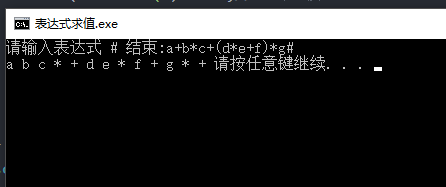2.2）实例

规则很多，还是用实例比较容易说清楚整个过程。以上面的转换为例，输入为a + b * c + (d * e + f)*g，处理过程如下：

1）首先读到a，直接输出。

2）读到“+”，将其放入到栈中。

3）读到b，直接输出。

此时栈和输出的情况如下：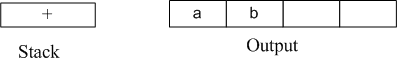4）读到“*”，因为栈顶元素"+"优先级比" * " 低，所以将" * "直接压入栈中。

5）读到c，直接输出。

此时栈和输出情况如下：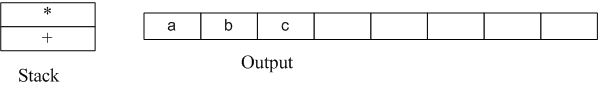6）读到" + "，因为栈顶元素" * "的优先级比它高，所以弹出" * "并输出， 同理，栈中下一个元素" + "优先级与读到的操作符" + "一样，所以也要弹出并输出。然后再将读到的" + "压入栈中。

此时栈和输出情况如下：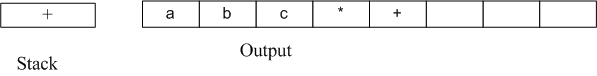7）下一个读到的为"("，它优先级最高，所以直接放入到栈中。

8）读到d，将其直接输出。

此时栈和输出情况如下：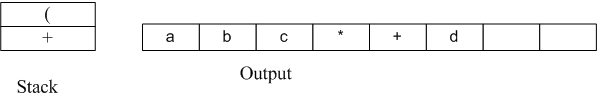9）读到" * "，由于只有遇到" ) "的时候左括号"("才会弹出，所以" * "直接压入栈中。

10）读到e，直接输出。

此时栈和输出情况如下：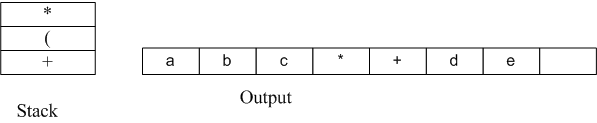11）读到" + "，弹出" * "并输出，然后将"+"压入栈中。

12）读到f，直接输出。

此时栈和输出情况：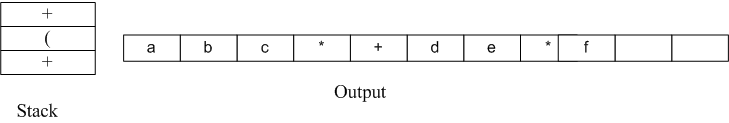13）接下来读到“）”，则直接将栈中元素弹出并输出直到遇到"("为止。这里右括号前只有一个操作符"+"被弹出并输出。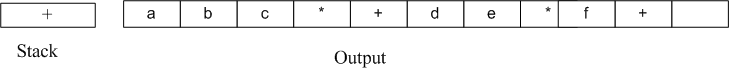14）读到" * "，压入栈中。读到g，直接输出。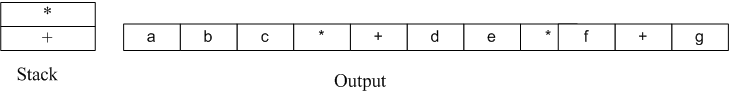15）此时输入数据已经读到末尾，栈中还有两个操作符“*”和" + "，直接弹出并输出。

至此整个转换过程完成。程序实现代码后续再补充了。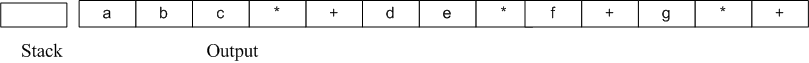2.3）转换的另一种方法

1)先按照运算符的优先级对中缀表达式加括号，变成( ( a+(b*c) ) + ( ((d*e)+f) *g ) )

2)将运算符移到括号的后面，变成((a(bc)*)+(((de)*f)+g)*)+

3)去掉括号，得到abc*+de*f+g*+


展开全文• 通常人在书写的时候习惯是书写中缀表达式，然而在计算机处理的时候中缀表达式的效率远小于后缀表达式，即操作数在前面，运算符在后面例如： 中缀表达式 A+B 后缀表达式AB+ A+B*C ABC*+ A*B+C*D AB*CD*+数据结构
• ## C语言中缀表达式求值(综合)

千次阅读 多人点赞 2019-04-13 10:59:06
题前需要了解的：中缀、后缀表达式是什么？（不知道你们知不知道，反正我当时不知道，搜的百度） 基本思路:先把输入的中缀表达式→后缀表达式→进行计算得出结果 栈：”先进先出，先进后出“！ 中缀转后缀（先把...C 数据结构
• 注：最主要需要注意的是代码中那个标注的getchar（）函数的使用 #include<stdio.h> #include<stdlib.h> #include<math.h> #define False 0 #define True 1 typedef struct ...ma数据结构 stack
• 后缀表达式求值c语言算法Here you will get algorithm and program for evolution of postfix expression in C. 在这里，您将获得用于C语言中后缀表达式演变的算法和程序。 In postfix or reverse polish notation...算法 堆栈 stack
• 数据结构c语言算术表达式求值（转化为后缀表达式的方法）（双栈：符号栈和数据栈）
• 实现数据结构中后缀表达式值c语言完整可运行代码。
• 一、先将中缀表达式转为后缀表达式 规则： 遇到数字：直接输出 遇到左括号：直接入栈 遇到右括号：输出栈顶元素，直至遇到左括号或者栈空；右括号不入栈 遇到运算符：分两种情况：i）当前运算符优先级大于栈顶...中缀表达式
• 数据结构 实验 数据类型浮点型 C语言 中缀表达式后缀 然后求值
• 在上一篇博客 栈应用：中缀表达式转后缀表达式 中我们知道如何通过栈将中缀表达式转为后缀表达式，这次我们继续用栈 来实现后缀表达式求值，结合上一篇博客。上一篇博客中是用c语言实现的，由于c语言中不支持模板...数据结构和算法 逆波兰表示法
• 大一菜鸟，初学编程，这是我的第一篇博客，希望能用博客记录我的成长之路。 初学数据结构，刚接触链表和栈，看到有中缀.../* 堆栈练习——中缀表达式转后缀表达式 */ #include #include #include #include<stdbool.h数据结构
• 表达式求值c语言版，能求出后缀，并根据后缀求表达式的值。仅供参考与交流
• ::iterator b = v.begin(); b < v.end();...}// 计算后缀表达式，仅支持加减乘除运算、操作数为非负整数的表达式。int postfix_eval(const char * postfix){const size_t N = strlen(postfix);if...
• 数据结构中表达式求值问题，将原表达式转换成后缀表达式C语言描述，VC环境下编译通过，运行正确。
• 说明 本程序支持括号以及小数计算 ...表达式求值可以借鉴该博文 我的代码 #include <stdio.h> #include <stdlib.h> #include <string.h> #define maxsize 100 #define N 7 typedef struct{/...算法
• 严蔚敏数据结构中表达式求值问题，将原表达式转换成后缀表达式C语言描述，VC环境下编译通过，运行正确。
• 后缀表达式的处理过程如下：扫描后缀表达式，凡遇操作数则将之压进堆栈，遇运算符则从堆栈中弹出两个操作数进行该运算，将运算结果压栈，然后继续扫描，直到后缀表达式被扫描完毕为止，此时栈底元素即为该后缀表...
• 我们平时数学上用的算术表达式（中缀表达式）转换为后缀表达式后，它主要是字符串形式（如果不会，建议先学习中转后的操作）。 要实现对其的求值，有如下几个步骤： （对整个字符串进行遍历） 将字符串型的数字转换...
• } //表达式运算符优先级低则执行出栈操作，弹出的栈顶元素成为后缀表达式的一部分 //当遇到“）”，则执行出栈操作直到“（”，但括号不计入后缀表达式中 while (!IsEmpty(Stack) && JudgeLevel(0, a[i]) (1, Stack...stack 数据结构
• c语言实现中缀表达式转后缀表达式并求得计算结果，用顺序栈结构。 当输入者输入错误信息的时候需要报错，并说明错误的种类。...

# c语言后缀表达式求值c语言 订阅